# Java Memory Model

- 13 mins## The problem

In Java, a program code can change a lot between its Java source code form, Byte code form, and machine code form. The Java source code focuses more on readability and clarity, while the machine code focuses on performance and efficiency. The JVM is allowed to optimize the code, with different degrees of optimization (depending on the compilation stage), as long as it remains correct. But, this task can be handy in the context of multi-threaded applications.

### Sequential consistency

There are multiple levels of caching while executing a program; the processor never operates on values directly in the main memory, but instead, it loads the values to its cache, manipulates them, then writes them back to the main memory. Let’s take the following example:

``````class Reorder {
int foo = 0;
int bar = 0;

void calc() {
foo += 1; //#1
bar += 1; //#2
foo += 2; //#3
}
}
``````

How the processor can execute the method `calc()` in memory?

1. Load `foo` from main memory to processor cache. Increment by 1, write it back the main memory (`#1`).
2. Load `bar` from main memory to processor cache. Increment by 1, write it back the main memory (`#2`).
3. Load `foo` from main memory to processor cache. Increment by 2, write it back the main memory (`#3`).

How the earlier example can be optimized ? by swiping the instructions (`#2` and `#3`):

``````void calc() {
foo+=1; //#1
foo+=2; //#3
bar+=1; //#2
}
``````
1. Load `foo` from main memory to processor cache. Increment by 1, Increment by 2, write it back the main memory (`#1` and `#3`).
2. Load `bar` from main memory to processor cache. Increment by 1, write it back the main memory (`#2`).

In a single-threaded program, this optimization can be considered without side effects, however, in a multi-threaded world, it introduces some abnormal behavior:

The possible values of the variables overtime in the two cases shows the slight difference:

• Before optimisation:
1. (foo == 0, bar == 0)
2. (foo == 1, bar == 0)
3. (foo == 1, bar == 1)
4. (foo == 3, bar == 1)
• After optimisation:
1. (foo == 0, bar == 0)
2. (foo == 1, bar == 0)
3. (foo == 3, bar == 0)
4. (foo == 3, bar == 1)

This previous example is an optimization that the JVM is allowed to do. The JVM can do much more complex optimizations, however, the outcome might be unexpected in a multi-threaded world! But why optimize then? The answer: memory access latency!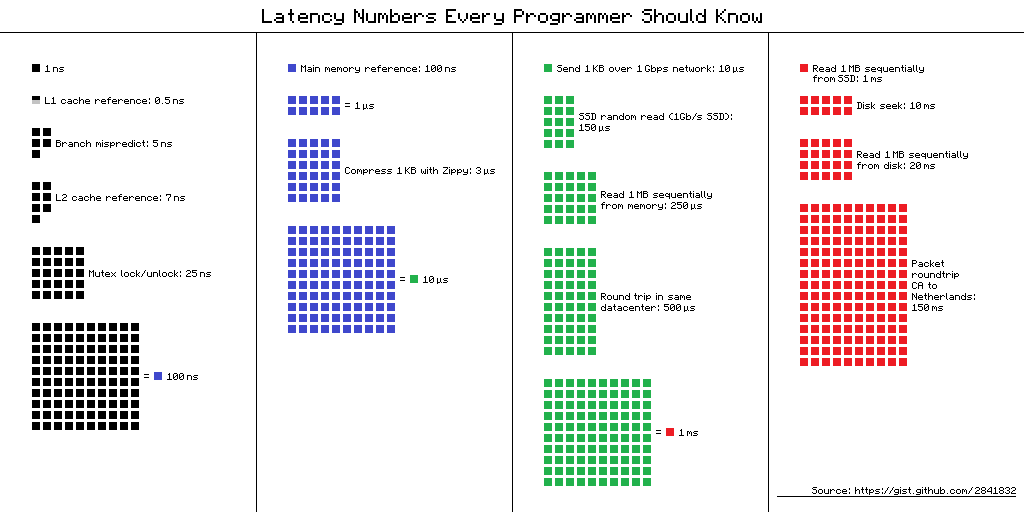### Eventual consistency

A machine can have multiprocessors, and (at some level) each processor has its cache, which means, each processor loads only the values it needs for its operations.
Lets we have two processors and the following program:

``````class Caching {
boolean flag = true;
int count = 0;

while (flag) count++;
}

flag = false;
}
}
``````

Let’s say processor `#1` will run the method `thread1()` and processor `#2` will run the method `thread2()`. An optimization can be the following:

• Since `thread1()` never modifies the `flag` variable, there is no need to load it from the main memory for each loop check, only once to the cache is enough -> the changes to `flag` might never be observed!
• Processor `#2` has no obligation to write it changes to the `flag` variable to the main memory! This means an optimization can be to simply not do the operation at all!

### 1.3 Atomicity

The atomicity in Java is to consider all values are atomic, which means that the modification to a variable (for example 64 bit types like `long` and `double`) to be done atomically.

Let’s consider the following example:

``````class LongTearing {
long foo = 0L;

foo = 0x0000FFFF; // 2147483647
}

foo = 0xFFFF0000; // -2147483648
}
}
``````

A 64 bit `long` variable, is written in two slots in the case of 32 memory, a problem can occur here:

• `thread1()` writes the first half of its value to memory `0000`.
• `thread2()` writes the second half of its value to memory `0000`.
• `thread1()` writes the second half of its value to memory `FFFF`.
• `thread1()` writes the first half of its value to memory `FFFF`.
• The final value of the variable will be then: `0xFFFFFFFF` !!!

### Processor optimization

Ordering operations sometimes are tied to the processor architecture. Optimization needs can be different for example between ARM processors and x86 processors. ARM processors can be more aggressive because they are designed for energy-consuming efficiency, than x86 processors which are more about calculation speed.

## What is the Java memory model?

Java memory model answers the question: what values can be observed upon reading from a specific field?

Formally specified by breaking down a Java program into actions and applying several orderings to these actions. If one can derive a so-called happens-before ordering between write actions, and a read actions of one field, the Java memory model guarantees that the read returns a particular value.

The Java memory machine guarantees intra-thread consistency equivalent to sequential consistency.

### Building blocks

According to the Java memory model, using the following keywords, a programmer can indicate to the JVM to refrain from optimizations that could otherwise cause concurrency issues:

• Field-scoped: `final`, `volatile`.
• Method-scoped: `synchronized` (method/block), `java.util.concurrent .*`.

In terms of the Java memory model, the above concepts introduce additional synchronization actions which introduce additional (partial) orders. Without such modifiers, reads and writes might not be ordered what results in a data race.
A memory model is a trade-off between a language’s simplicity (consistency/atomicity) and its performance.

### Volatile

Lets take the following example:

``````class DataRace {

}

}
}
``````

The lines `#1` and `#2` can be reordered! This means, the assertion in method `thread1()` can fail in a multi-threaded world!
A solution ? The keyword `volatile`:

``````class DataRace {

}

}
}
``````

`volatile` implies for two threads with a write-read relationship on the *same field*, certain optimizations are not allowed!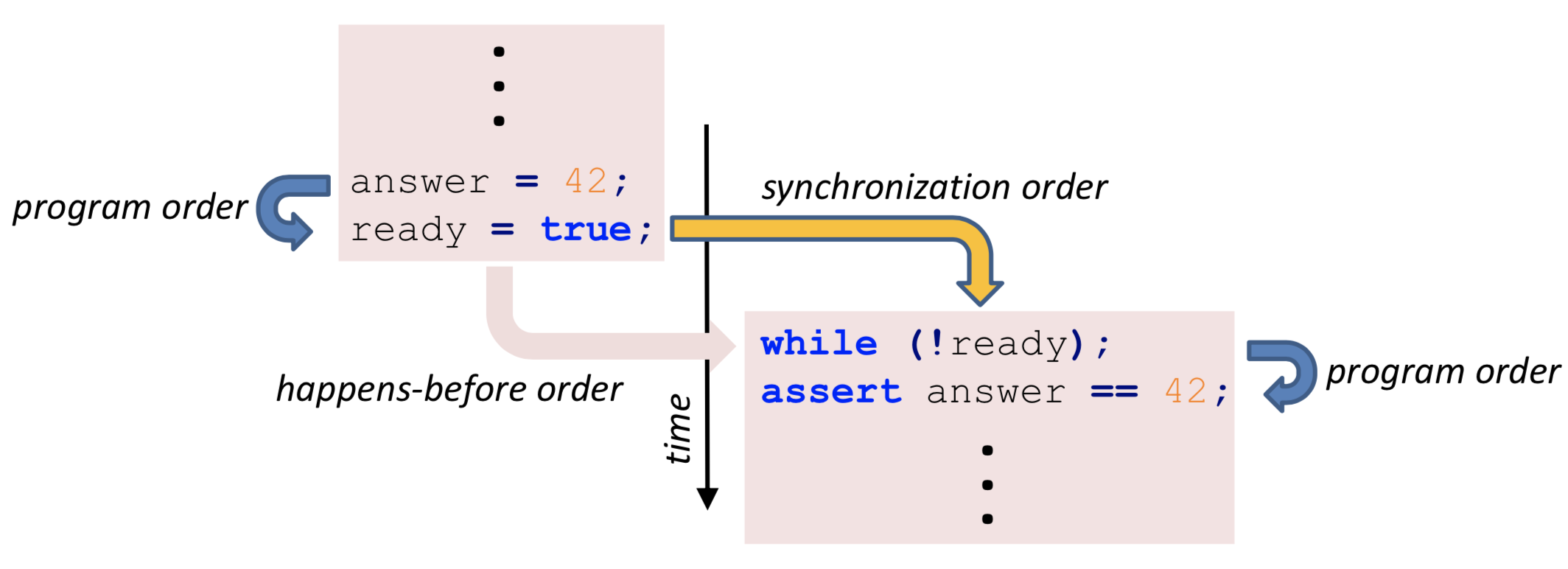1. When a thread writes to a `volatile` variable, all of its previous writes are guaranteed to be visible to another thread when that thread is reading the same value.
2. Both threads must align “their” `volatile` value with that in main memory (flush).
3. If the `volatile` value was a `long` or a `double` value, word-tearing was forbidden.

### Synchronized

Another way to achieve the synchronization is by using: `synchronized` Lets check the following example assuming the second thread acquires the lock first:

``````class DataRace {

}

synchronized void thread2() { //Assuming this is called 1st
}
}
``````

When a thread releases a monitor, all of its previous writes are guaranteed to be visible to another thread after that thread is locking the same monitor.. This only applies for two threads with an *unlock-lock relationship* on the same monitor!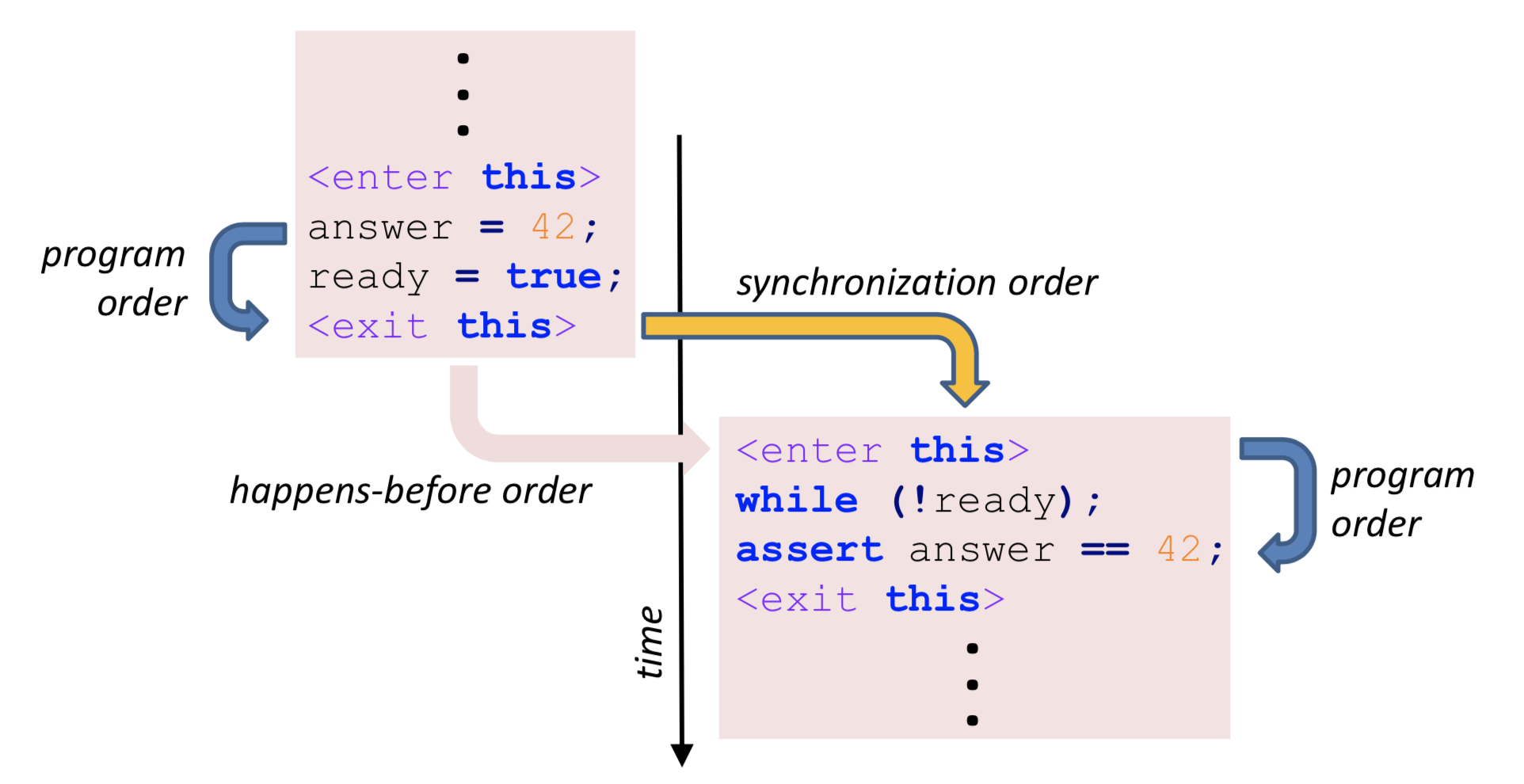``````class ThreadLifeCycle {
int foo = 0;

void method() {
foo = 42;
@Override
public void run() {
assert foo == 42;
}
}.start();
}
}
``````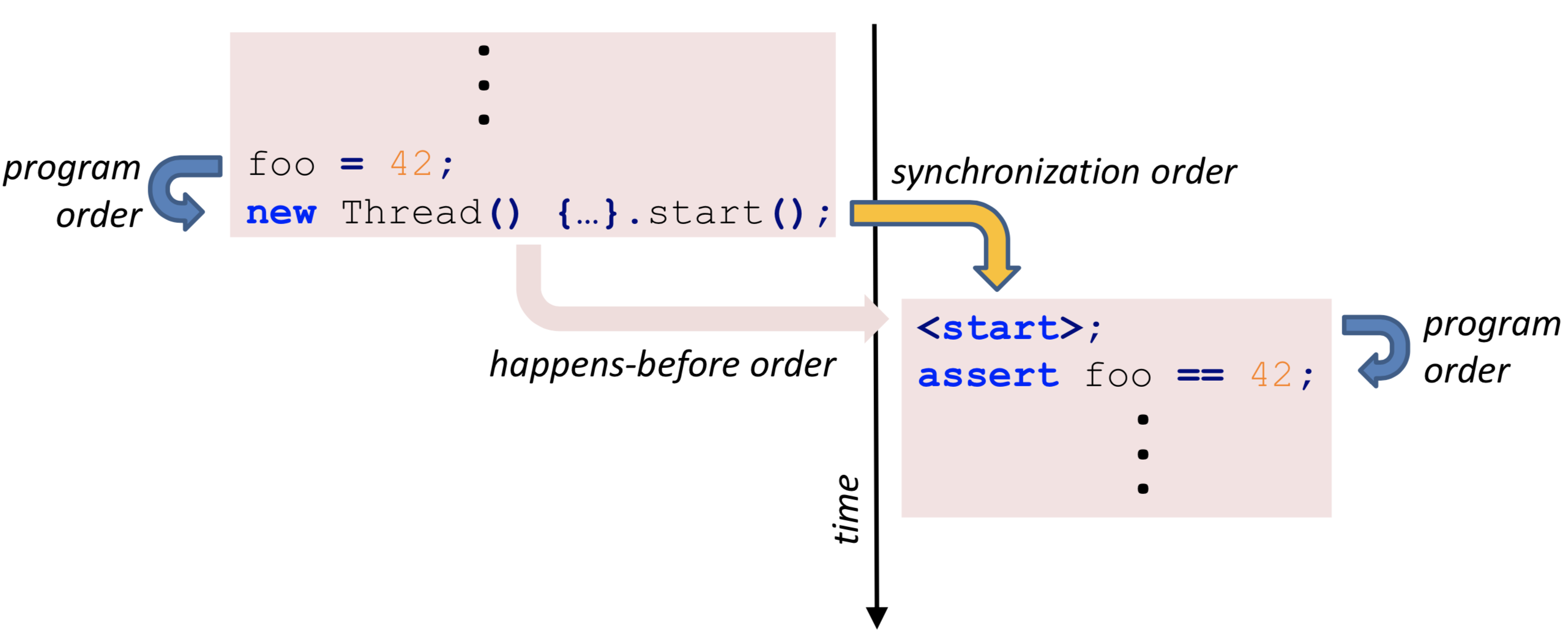Similarly, a thread that joins another thread is guaranteed to see all values that were set by the joined thread.

### Final field semantics

When a thread creates an instance, the instance’s `final` fields are frozen. The Java memory model requires a field’s initial value to be visible in the initialized form to other threads.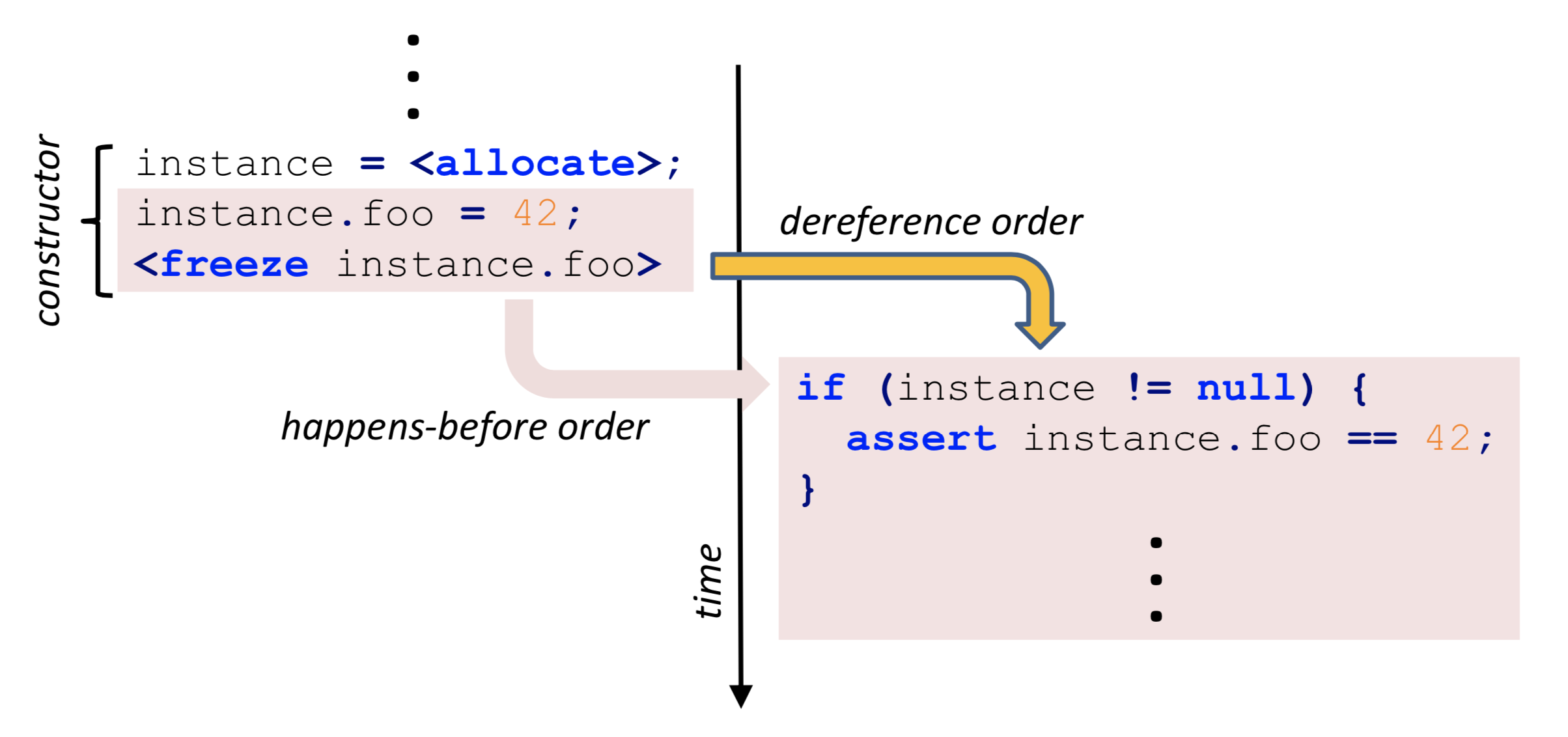This requirement also holds for properties that are dereferenced via a `final` field, even if the field value’s properties are not final themselves (memory-chain order).

### External actions

A JIT-compiler cannot determine the side-effects of a native operation. Therefore, external actions are guaranteed to not be reordered.

``````class Externalization {
int foo = 0;

void method() {
foo = 42;
jni(); // Not re-ordered
}

native void jni();
}
``````

External actions include JNI, socket communication, file system operations, or interaction with the console ( non-exclusive list).

Thread-divergence actions are guaranteed to not be reordered. This prevents surprising outcomes of actions that might never be reached.

``````class ThreadDivergence {
int foo = 42;

while (true) ;
foo = 0; // Not re-ordered
}

assert foo == 42;
}
}
``````

In the previous example, in the method `thread1()` the line `foo = 0` is unreachable. Thus not re-ordered.

## In Practice

The following are some practical examples of Java Memory Model use (or misuse).

### Double-checking

The following is a lazy instance creation example:

``````class DoubleChecked {
static volatile DoubleChecked instance;

static DoubleChecked getInstance() {
if (instance == null) {
synchronized (DoubleChecked.class) {
if (instance == null) {
instance = new DoubleChecked();
}
}
}
return instance;
}

int foo = 0;

DoubleChecked() {
foo = 42;
}

void method() {
assert foo == 42;
}
}
``````

This example works because of `volatile`, omitting it may cause having an instance of an object created, but uninitialized!

### Arrays

Declaring an array to be `volatile` does not make its elements `volatile`! In the following example, there is no write-read edge because the array is only read by any thread:

``````class DataRace {
volatile boolean[] ready = new boolean[]{false};

For such volatile element access: `java.util.concurrent.atomic.AtomicIntegerArray`.